新竹統一馬武督渡假村 Uni-Resort

3,980

臨近：

飯店位置： 關西鎮 客房數： 73

選擇專案房型及間數

更改 新竹統一馬武督渡假村 的入住日期

更改 新竹統一馬武督渡假村 的空房情形及價格

入住日期 停留夜數 退房日期
•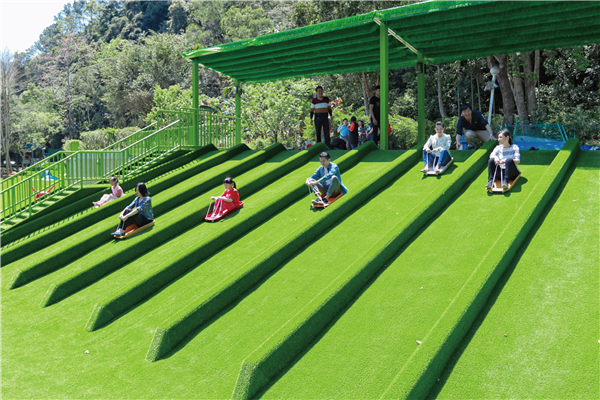遊樂場
•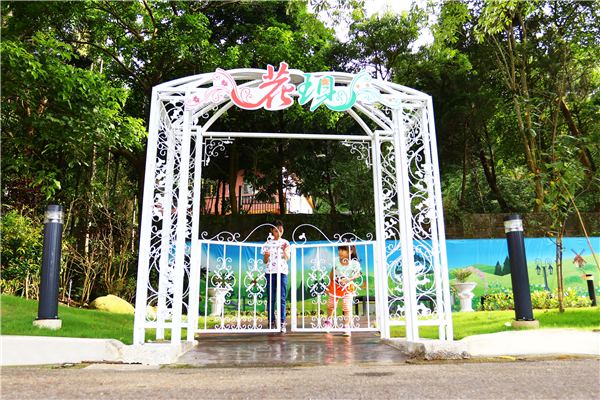入口
•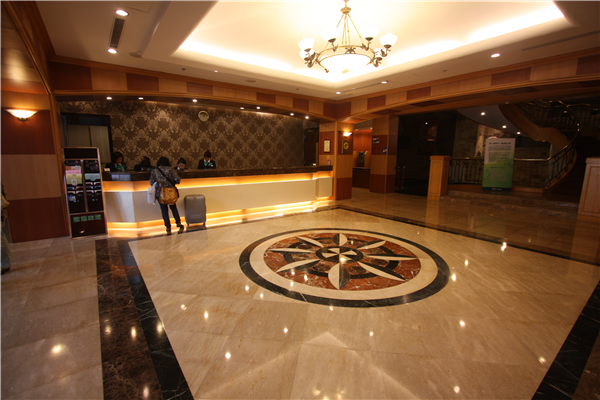大廳
•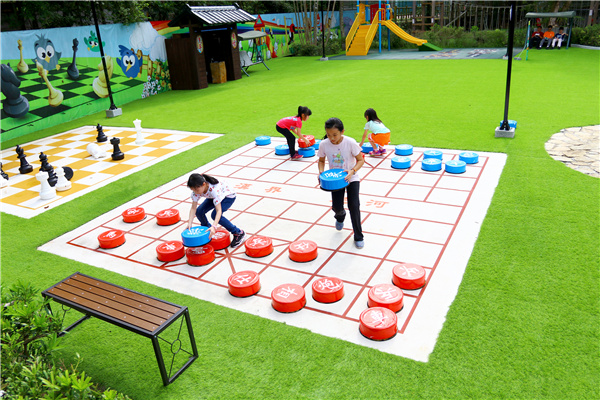幸福草原
•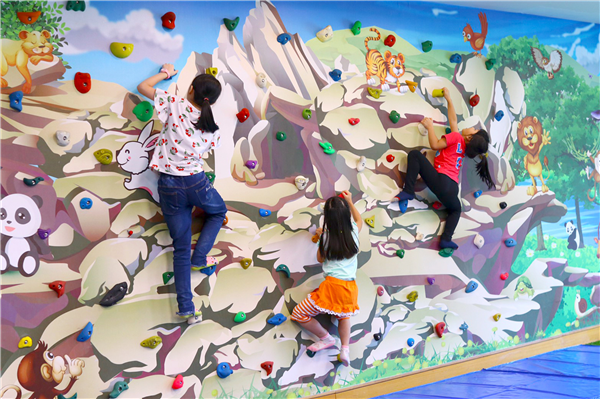兒童俱樂部
•手搖船
•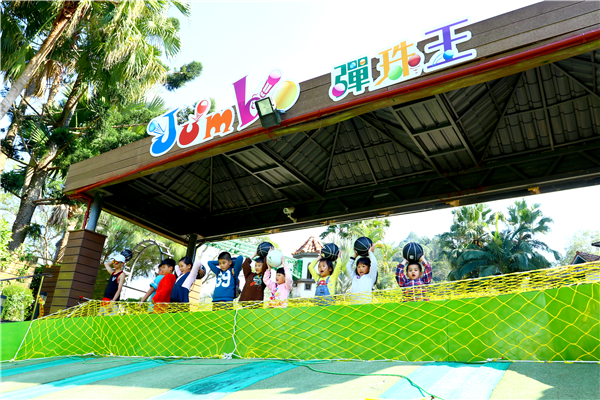娛樂設施
•娛樂設施
•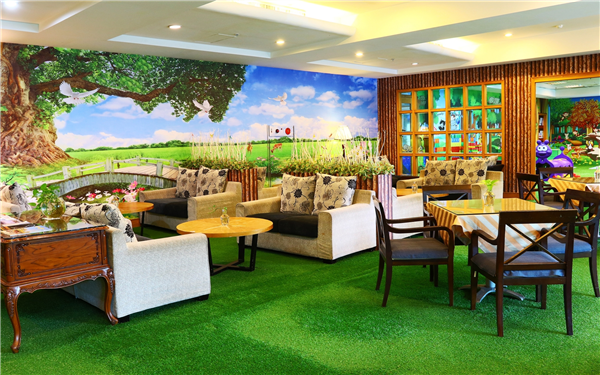兒童俱樂部
•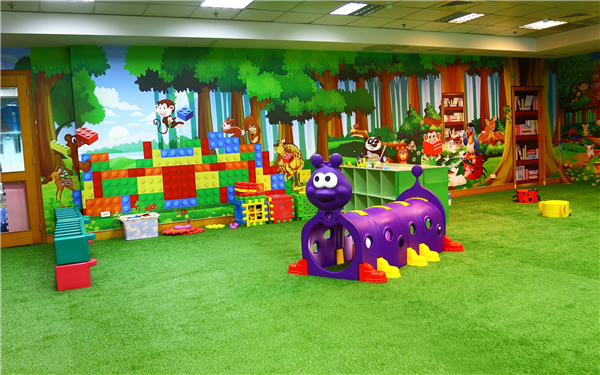兒童俱樂部
•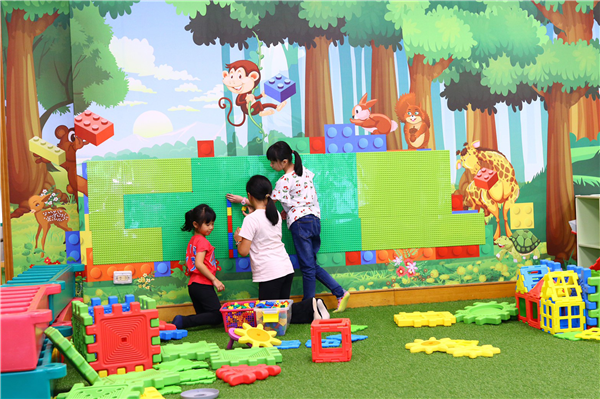兒童俱樂部
•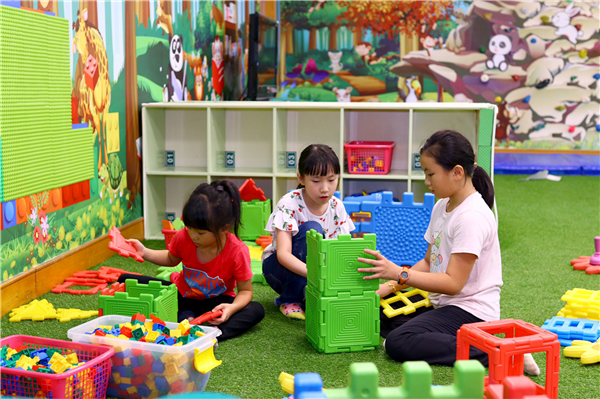娛樂設施
•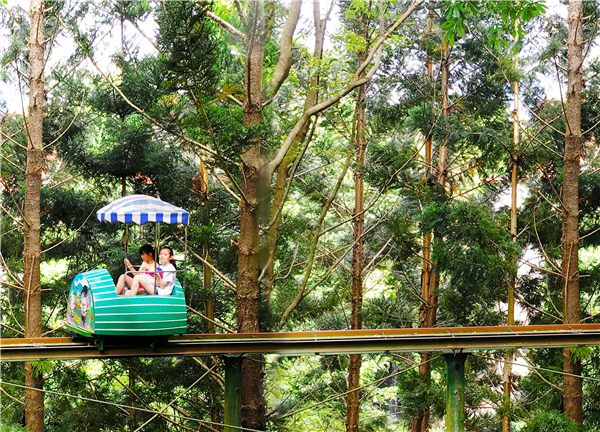娛樂設施
•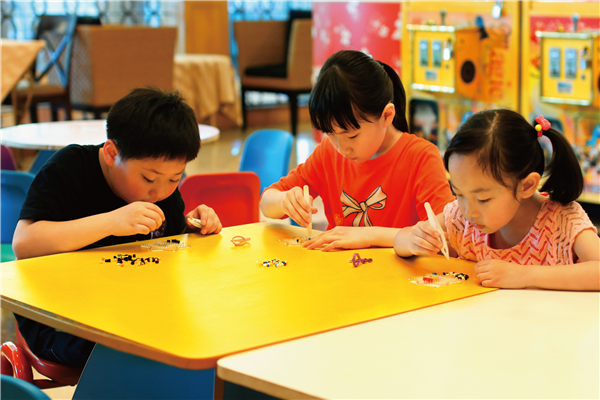娛樂設施-DIY
•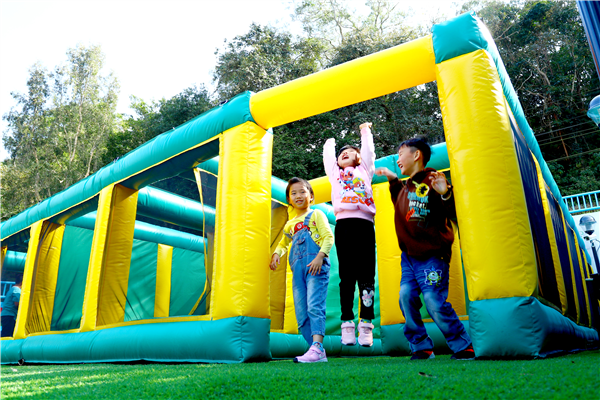娛樂設施-Fun遊迷宮
•游泳池
•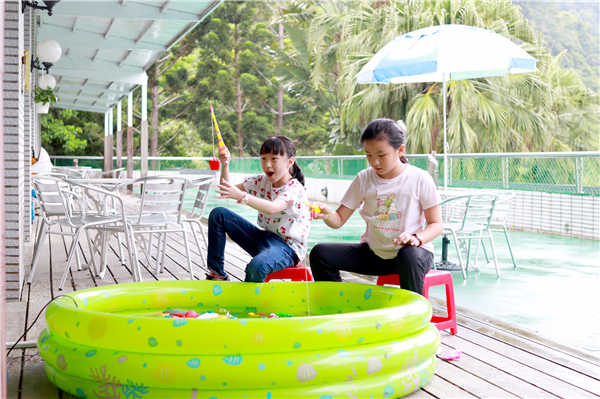娛樂設施
•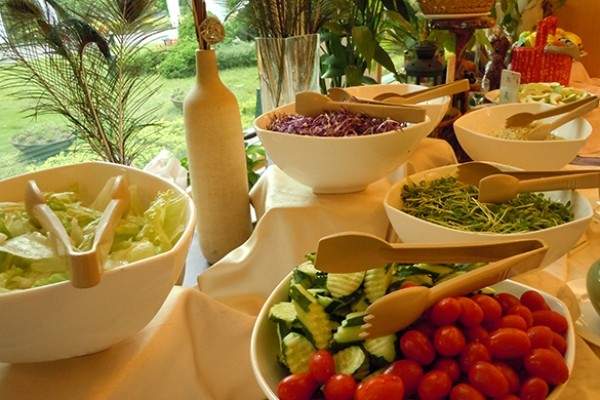環境
•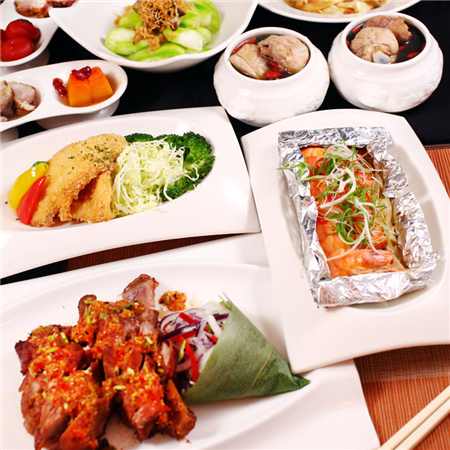餐廳
•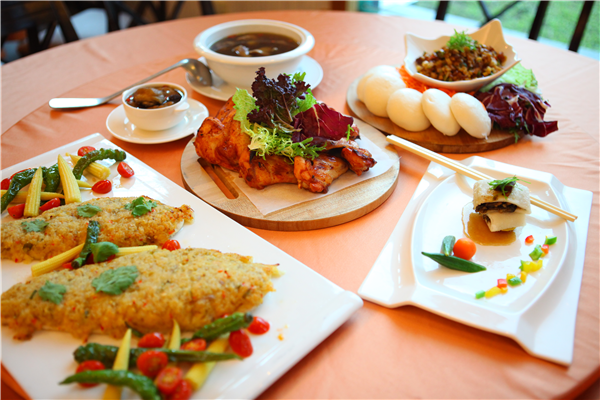餐廳
•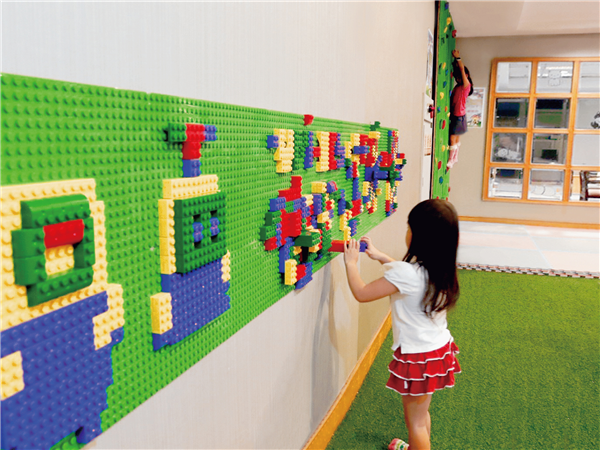酒店內部
•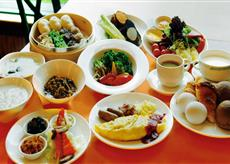餐廳
•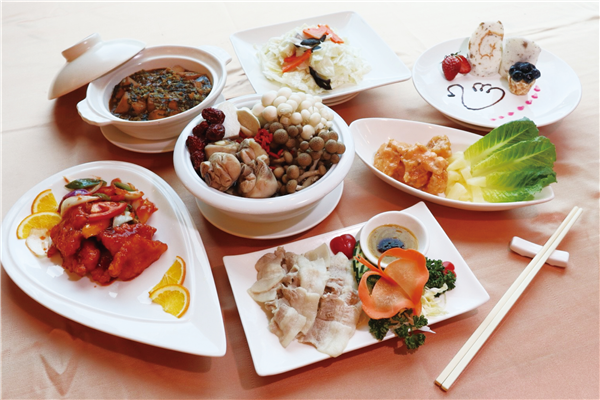餐廳
•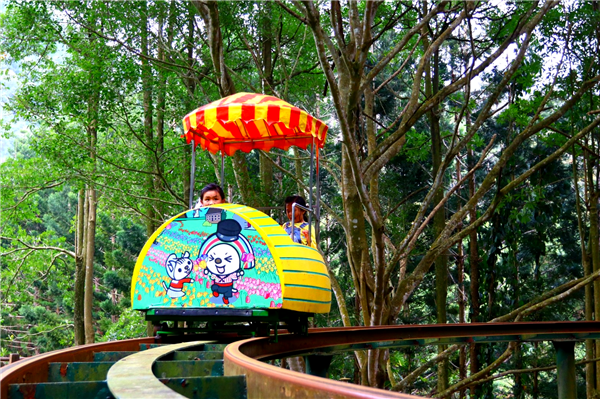娛樂設施
•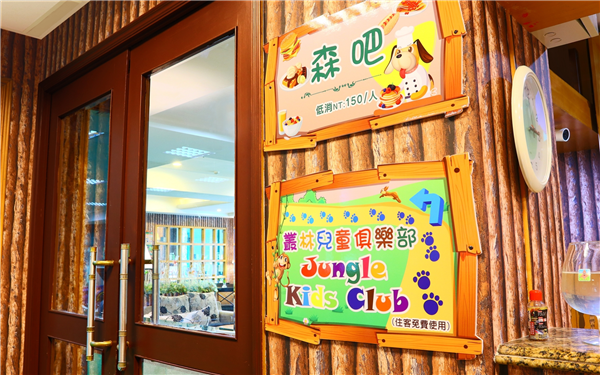兒童俱樂部
•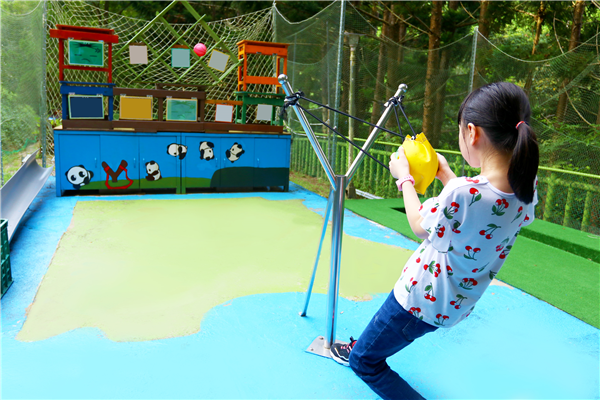娛樂設施
•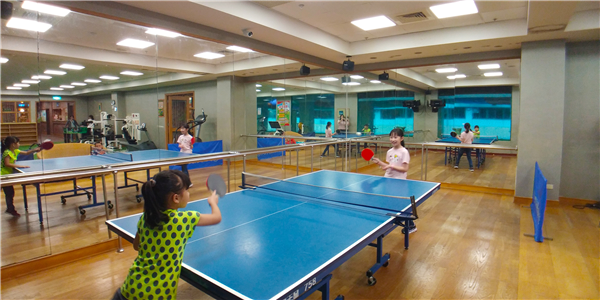桌球
•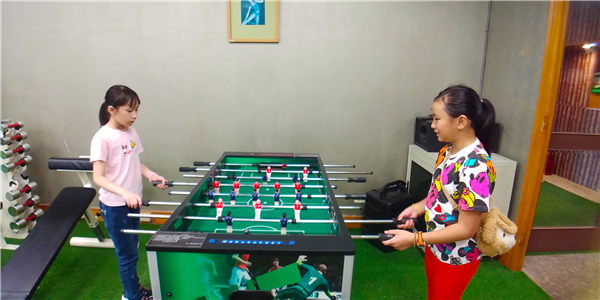兒童俱樂部-手足球
•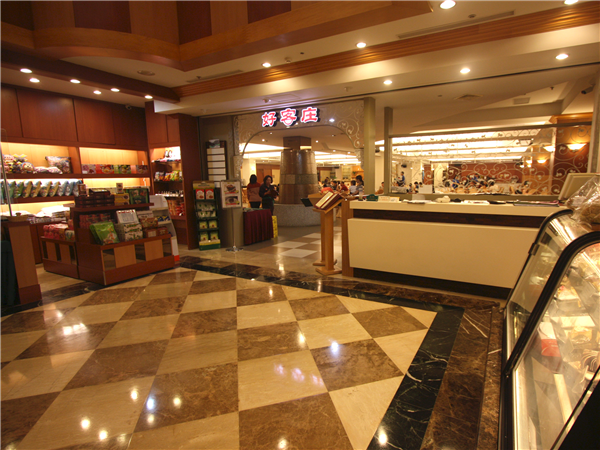餐廳
•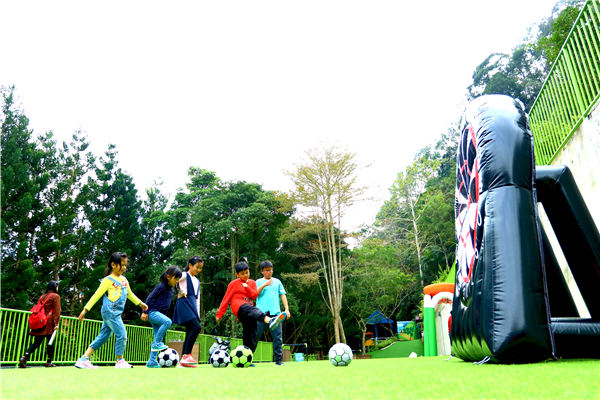娛樂設施
•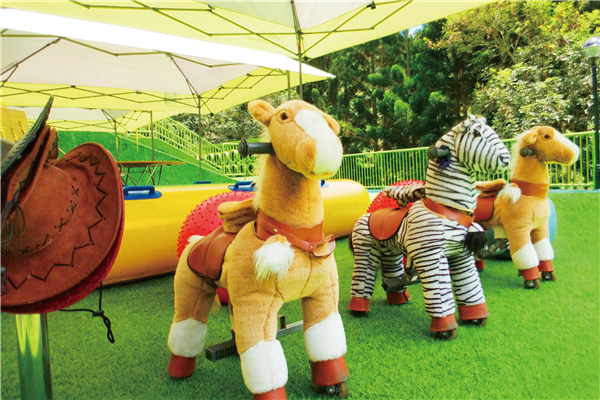娛樂設施
•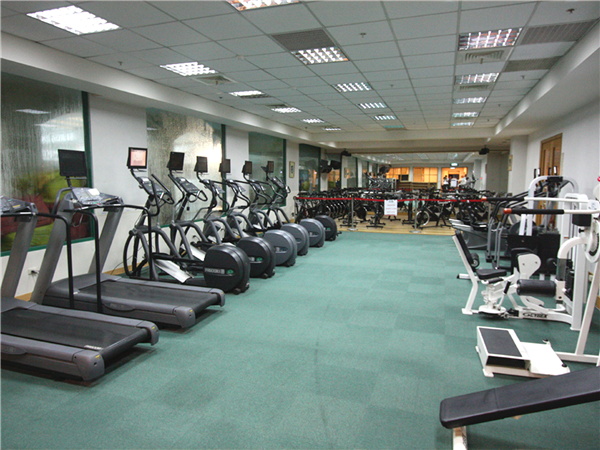健身房
•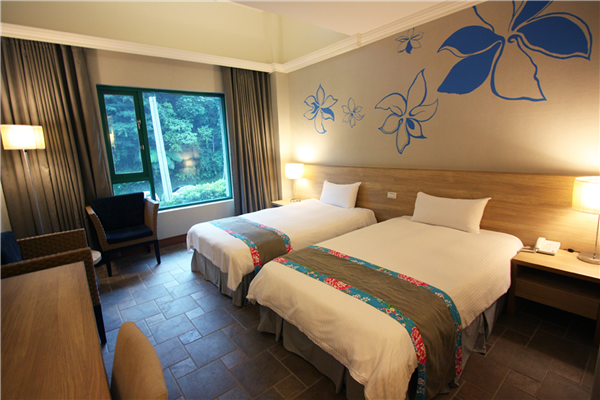客房
•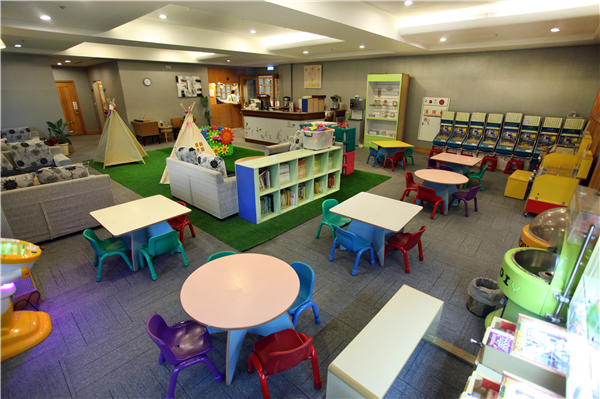酒店內部
•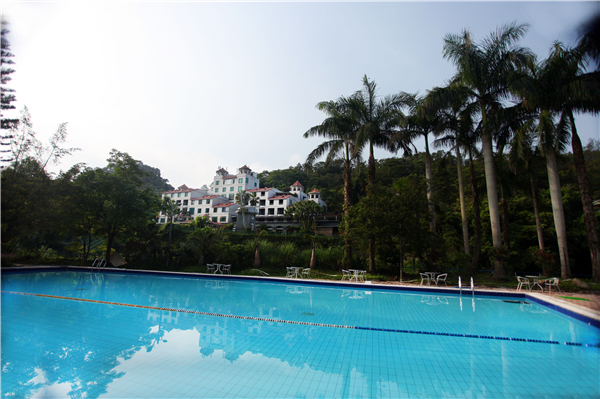游泳池
•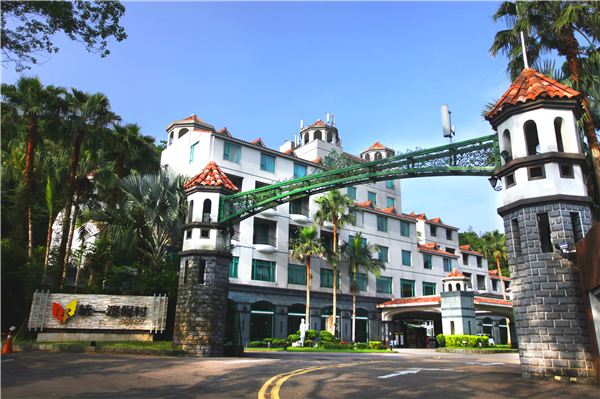入口
•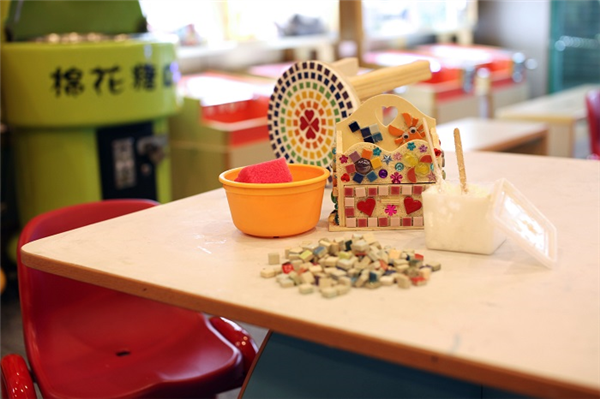兒童俱樂部
•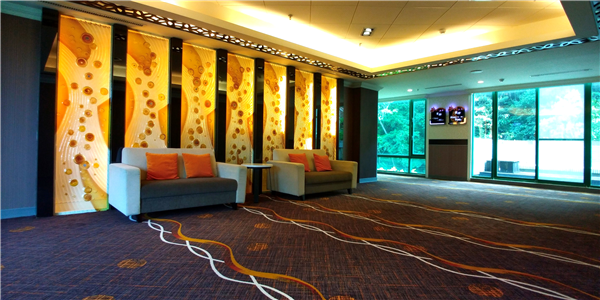酒店內部-梯廳
•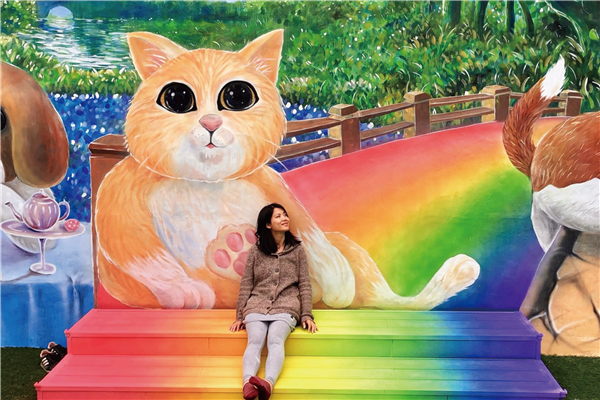環境
•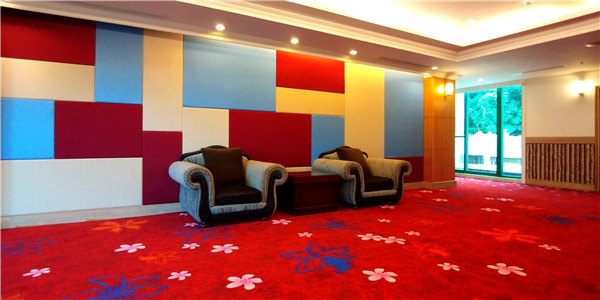酒店內部-梯廳
•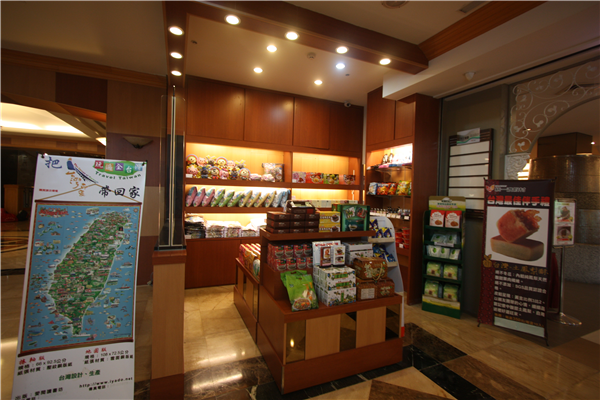商店
•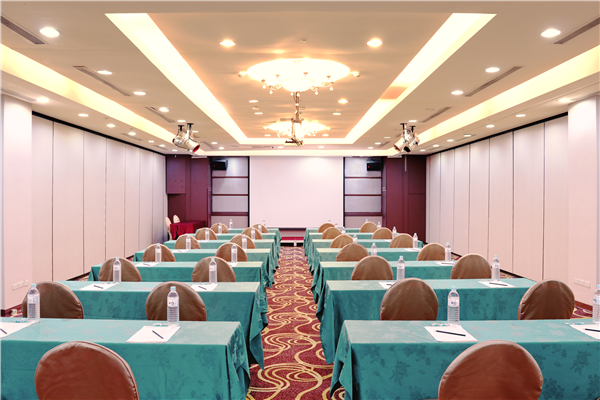會議室
•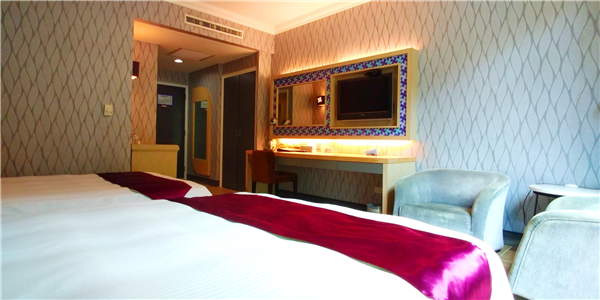客房
•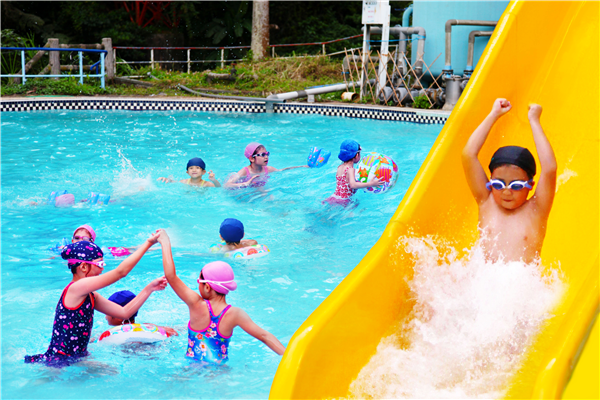游泳池
•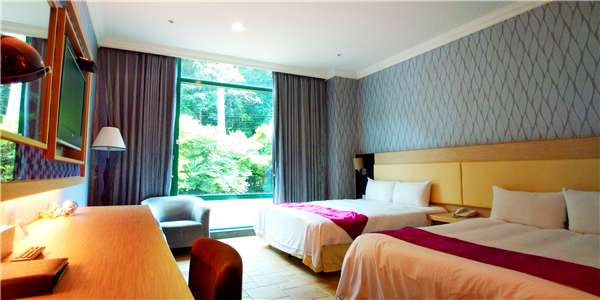客房
•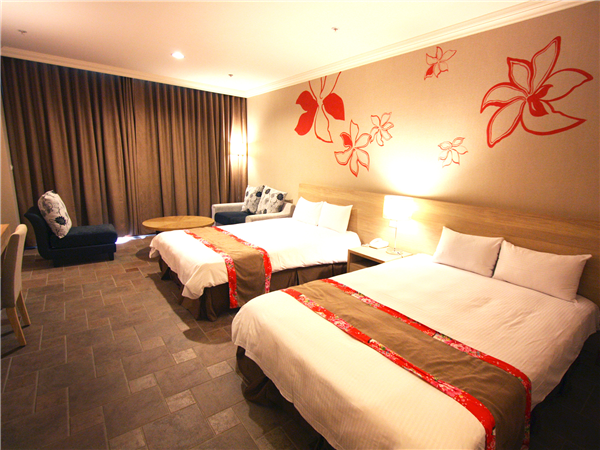客房
•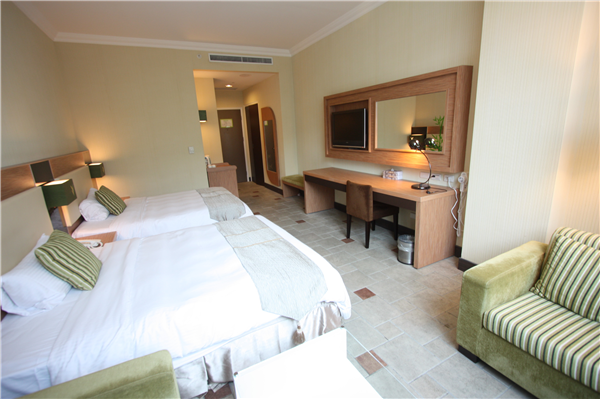客房
•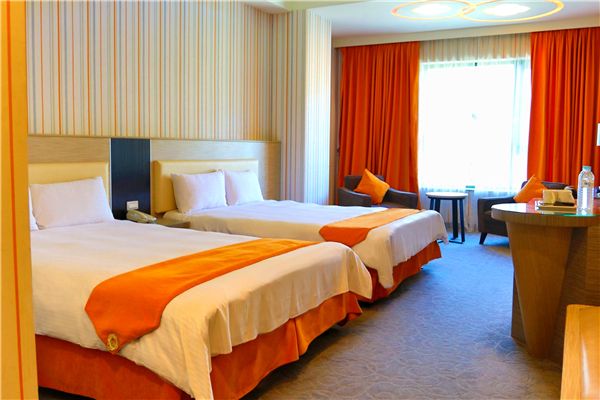客房
•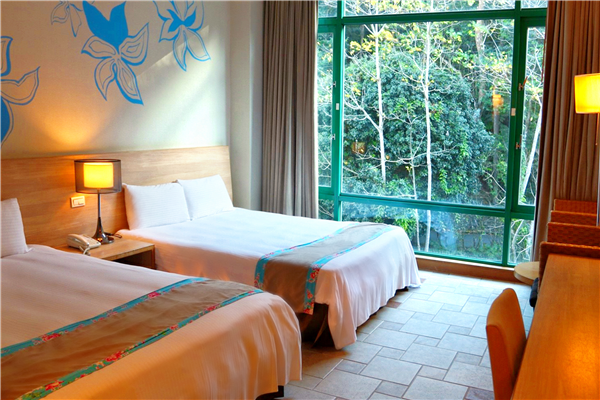客房
•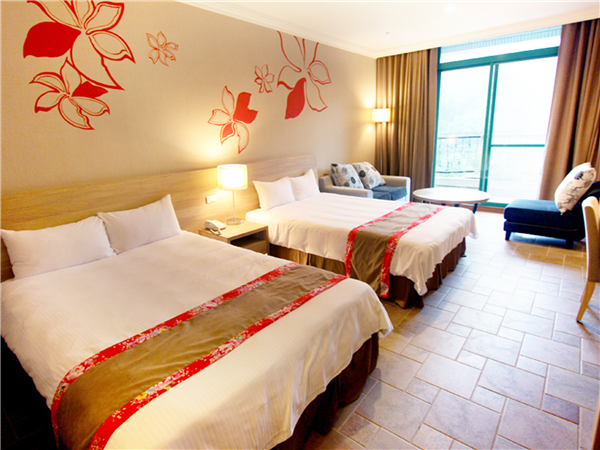客房
•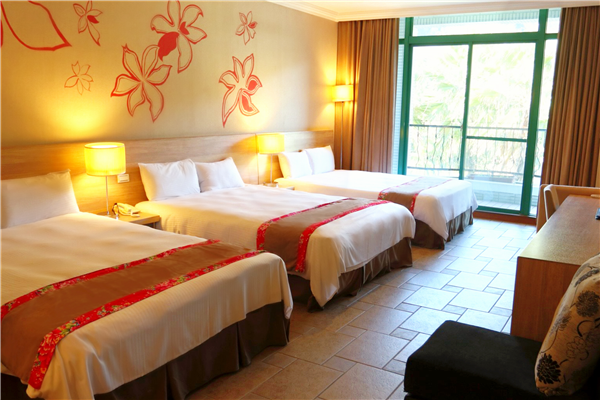客房
•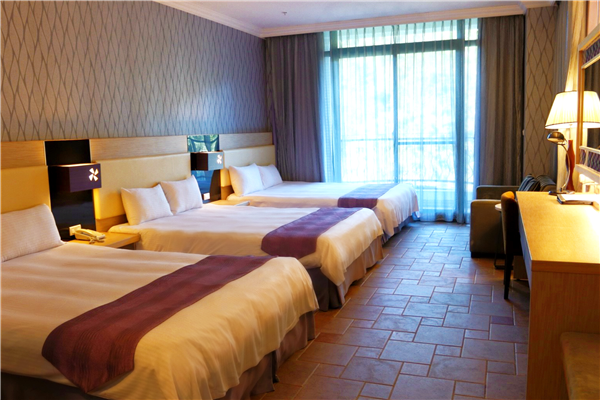客房
•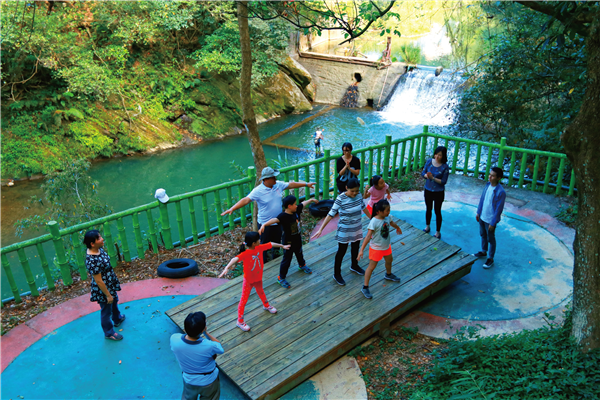遊樂場
•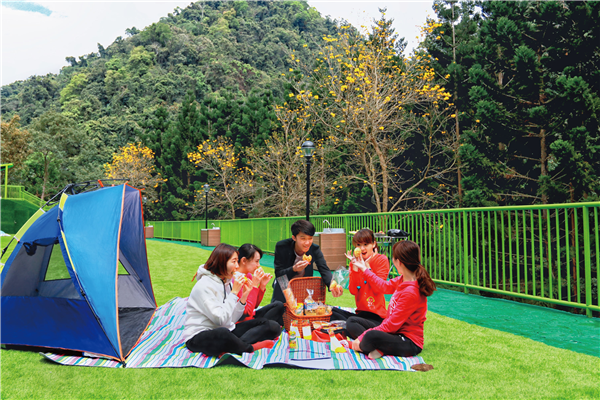遊樂場
•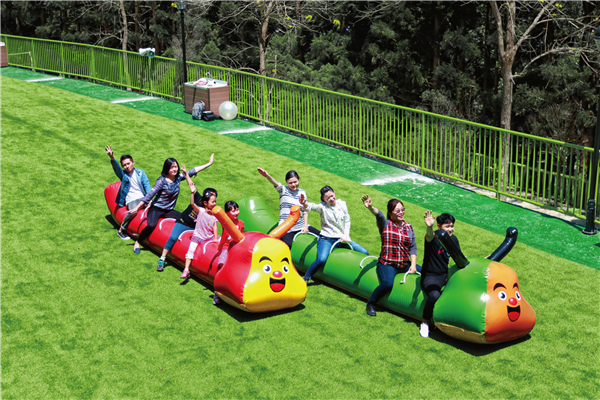遊樂場
•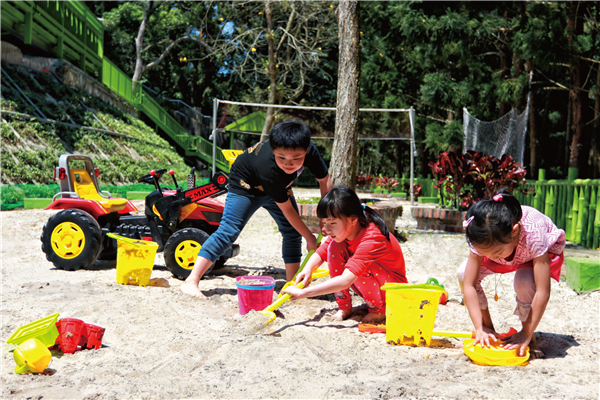遊樂場
•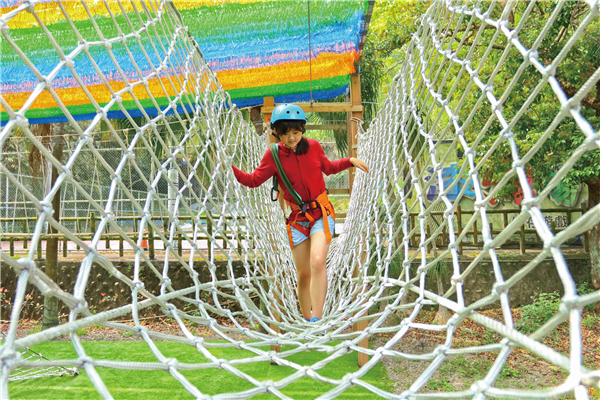遊樂場
•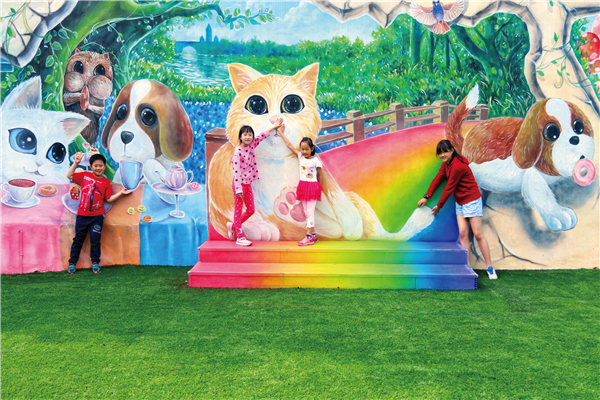遊樂場
•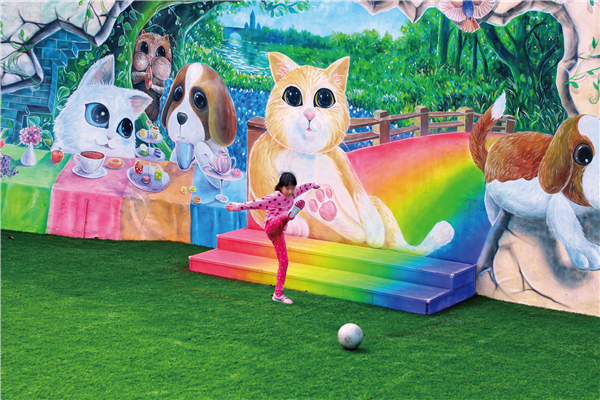遊樂場
•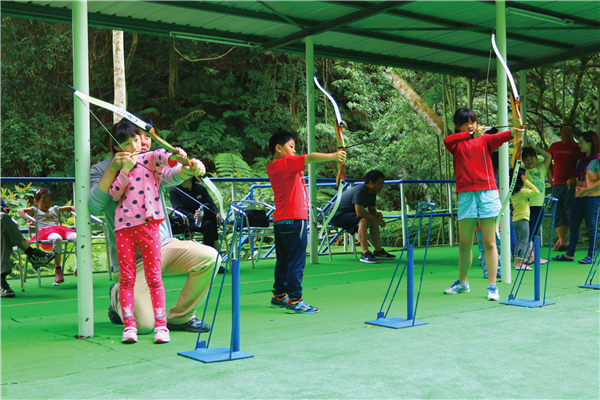遊樂場
•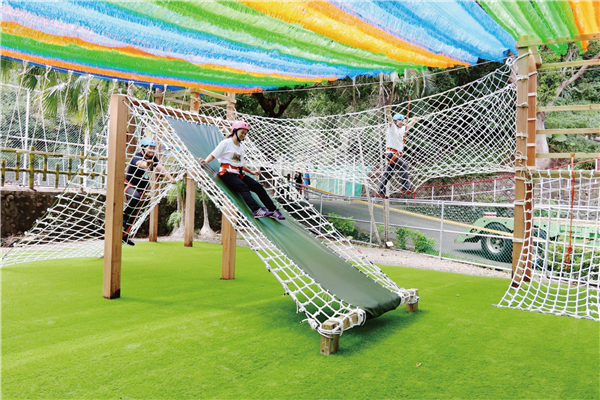遊樂場
•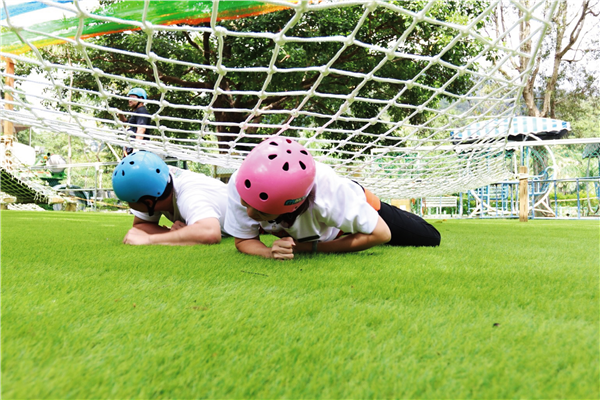遊樂場
•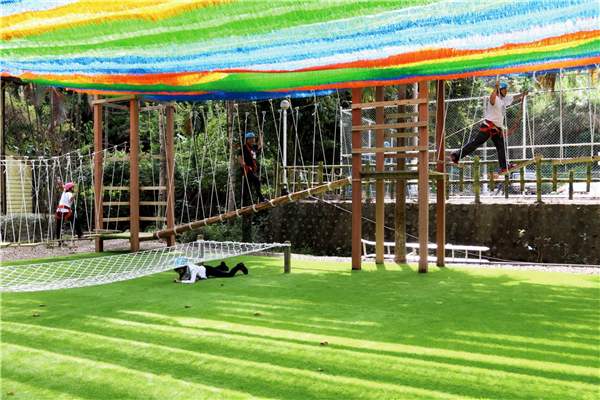遊樂場

飯店設有　 飯店未設 飯店設施

飯店設施
• 24小時客房服務
• 商務中心
• 洗衣服務/乾洗
• Wi-Fi in public areas
• 夜總會
• 禮賓接待服務
• 代客泊車
• 娛樂場
• 自行車出租服務
• 保管服務
• 客房服務
• 行政樓層
• 停車場
• 家庭房
• 酒吧
• 兒童看護服務
• 接送服務
• 電梯
• 可帶寵物
• 旅遊服務
• 飯店/機場接送
• 吸煙房
• 會議設施
• 餐廳
• 咖啡店
• 殘障人士設施
• 髮廊
• 商店
• 池畔吧
房間設施
• DVD/CD播放器
• 小廚房
• 無線上網(收費)
• 休憩區
• 微波爐
• 燙衣板
• 免費瓶裝水
• 房內保險箱
• 獨立淋浴間
• 公共浴室
• 房內遊戲機
• 獨立用餐區
• 冰箱
• 手提電腦保險箱
• 禁煙房
• 冷氣
• 按摩浴缸
• 私人泳池
• 加長的床(>2m)
• 日報
• 茶與咖啡沖泡設備
• 可提供相連房間
• 書桌
• 衛星頻道/有線電視
• 吹風機
• 洗衣機
• 迷你吧
• 寬頻上網
• 浴室
• 陽台/露台
• 寬頻上網(免費)
• 浴缸
• 電影點播服務
• 寬頻上網(收費)
• 浴袍
• 電視
• 寬頻上網(無線)
• 液晶電視
• 電風扇
• 尊用休息室
• 無線上網(免費)
運動&娛樂設施
• spa
• 三溫暖/水療設備
• 網球場
• 健身房/健身設施
• 水上活動
• 花園
• 兒童娛樂室
• 水上運動項目(非機動項目)
• 蒸浴室
• 壁球場
• 溫泉
• 遊戲室/娛樂場
• 室內游泳池
• 游泳池(兒童)
• 高爾夫球場(3km內)
• 室外游泳池
• 漩渦按摩浴池
• 高爾夫球場(飯店)
• 按摩
• 私人海灘

飯店簡介

【特色說明】
．環　　境：長壽之鄉
．自然環境：背倚擋耙山，橫跨馬武督溪。
．主題功能：渡假會議中心。
．餐飲特色：客家鄉土料理。
．建築風格：西班牙。
．住宿特色：多樣的住宿選擇，面溪客房。
．鄰近景點：馬武督探索森林公園、六福村主題樂園、金勇蕃茄園、高爾夫球場。
．特色設施：山泉水游泳池、高空踏踏樂、 滑草場、渡假會議中心、抗壓舒活區、DIY教室、射箭場、綜合球場、戶外滑水道、兒童戲水池、室內溫水游泳池、室內桌撞球。

飯店條款

兒童及加床需求：
• 加床政策根據您所選定的客房而有所不同，更多詳情請查看您所選定的客房的政策

相關資訊

退房時間 11:00 AM 110V Yes Yes 73間
縮小搜尋結果範圍
0
0